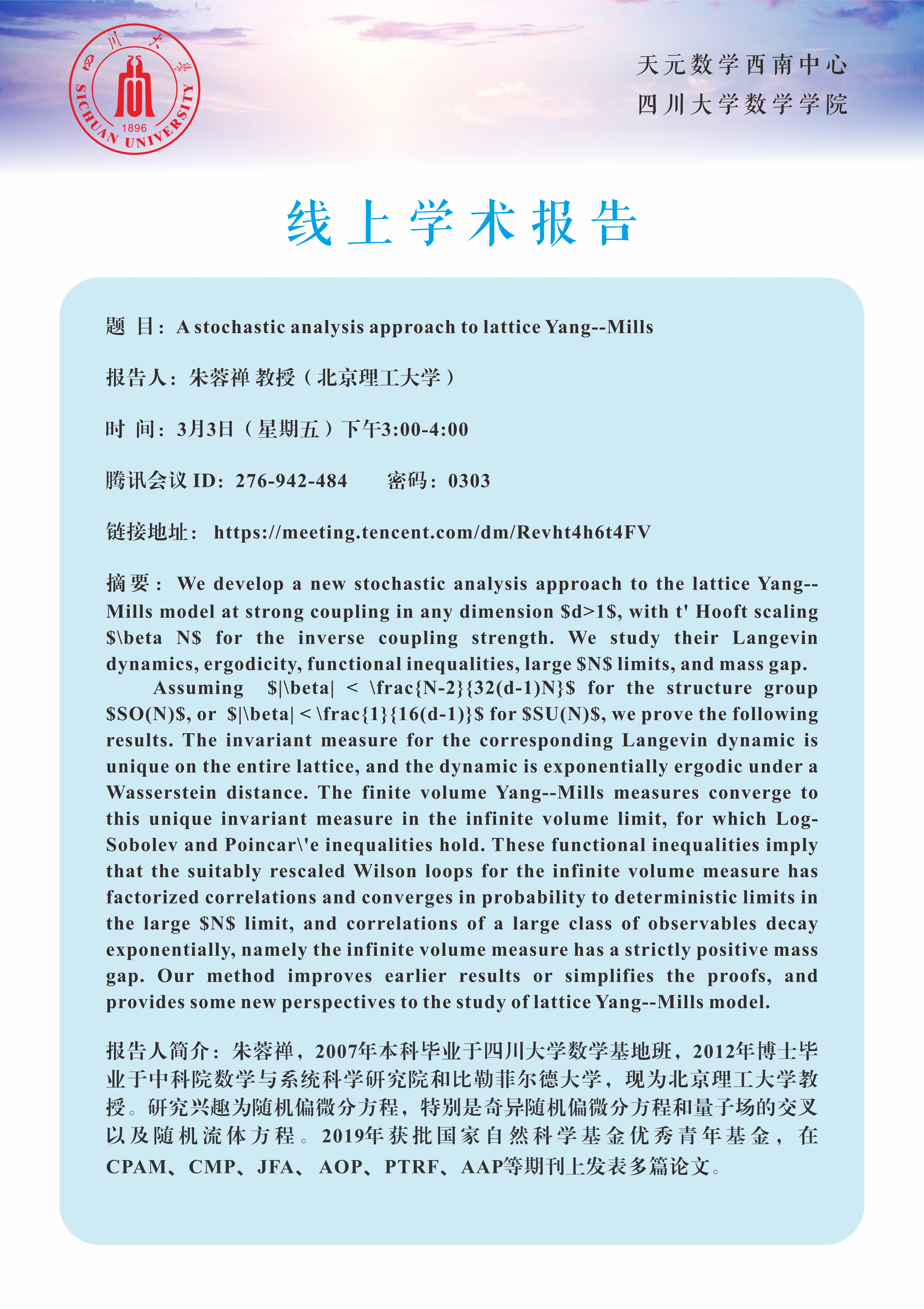A stochastic analysis approach to lattice Yang--Mills

We develop a new stochastic analysis approach to the lattice Yang--Mills model at strong coupling in any dimension $d>1$, with t' Hooft scaling $\beta N$ for the inverse coupling strength. We study their Langevin dynamics, ergodicity, functional inequalities, large $N$ limits, and mass gap.

Assuming  $|\beta| < \frac{N-2}{32(d-1)N}$ for the structure group  $SO(N)$, or  $|\beta| < \frac{1}{16(d-1)}$ for $SU(N)$, we prove the following results. The invariant measure for the corresponding Langevin dynamic is unique on the entire lattice, and the dynamic is exponentially ergodic under a Wasserstein distance. The finite volume Yang--Mills measures converge to this unique invariant measure in the infinite volume limit, for which Log-Sobolev and Poincar\'e inequalities hold. These functional inequalities imply that the suitably rescaled Wilson loops for the infinite volume measure has factorized correlations and converges in probability to deterministic limits in the large $N$ limit, and correlations of a large class of observables decay exponentially, namely the infinite volume measure has a strictly positive mass gap. Our method improves earlier results or simplifies the proofs, and provides some new perspectives to the study of lattice Yang--Mills model.